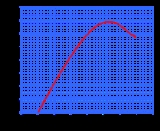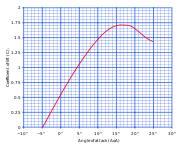xZero lift axisEncyclopediaWhen symmetric aerofoils are moving parallel to the chord line
Chord (aircraft)
In aeronautics, chord refers to the imaginary straight line joining the trailing edge and the center of curvature of the leading edge of the cross-section of an airfoil...

of the aerofoil, zero lift
Lift (force)
A fluid flowing past the surface of a body exerts a surface force on it. Lift is the component of this force that is perpendicular to the oncoming flow direction. It contrasts with the drag force, which is the component of the surface force parallel to the flow direction...

is generated. However, when cambered
Camber (aerodynamics)
Camber, in aeronautics and aeronautical engineering, is the asymmetry between the top and the bottom surfaces of an aerofoil. An aerofoil that is not cambered is called a symmetric aerofoil...

aerofoils are moving parallel to the chord line, lift is generated. (See diagram at right.)

A cambered aerofoil generates no lift when it is moving parallel to an axis called the zero lift axis (or the zero lift line.) When the angle of attack
Angle of attack
Angle of attack is a term used in fluid dynamics to describe the angle between a reference line on a lifting body and the vector representing the relative motion between the lifting body and the fluid through which it is moving...

on an aerofoil is measured relative to the zero lift axis it is true to say the lift coefficient
Lift coefficient
The lift coefficient is a dimensionless coefficient that relates the lift generated by a lifting body, the dynamic pressure of the fluid flow around the body, and a reference area associated with the body...

is zero when the angle of attack is zero. For this reason, on a cambered aerofoil the zero lift line is better than the chord line when describing the angle of attack.

For symmetric aerofoils, the chord line and the zero lift line are the same.
The source of this article is wikipedia, the free encyclopedia.  The text of this article is licensed under the GFDL.